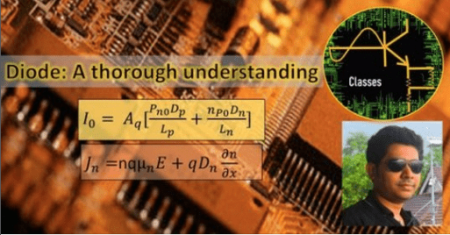﻿ Electronics _ Diode (Part 1) - A Solid State Approach » GFXhome WS

Information of news
23-06-2021, 20:16

### Electronics _ Diode (Part 1) - A Solid State Approach

Category: TutorialsCreated by Sumanta kumar Pal | Last updated 7/2019
Duration: 3h 13m | 7 sections | 34 lectures | Video: 1280x720, 44 KHz | 4.2 GB
Genre: eLearning | Language: English + Sub

==========

Diode as a Solid State Device-know what is happening inside the diode-Very useful for 2nd year Electronics Engg students

What you'll learn
Understand the fundamentals of P-N Junction Semiconductor diode by adopting a Solid State approach,Know what happens inside the diode under different conditions.
Analysis of Diode under Forward Bias,its Energy Band Diagram,Carrier Concentration variation w.r.t distance,Electric Field picture
Mathematical expressions for Electric potential, Depletion Layer under Equilibrium.
Discussion on Quasi-Fermi level and Energy Band Diagram plot.
Carrier concentration as a Function of distance under applied forward bias.
Current Density as a function of distance under applied forward bias.
A common misunderstanding-Cut in voltage VS Built in voltage.
V-I Characteristics of Diode under different bias conditions.
Understand Diode function Generator & Diode Transfer-function Generator.
Diode properties under reverse biased condition.
Discussion on Breakdown phenomena-Avalanche Breakdown & Zener Breakdown.
Show less

Requirements
Basic knowledge of science,High school mathematicsFundamental ideas of current, voltage and electric fieldKnowledge of Semiconductor Physics,Complete idea regarding Drift current,Diffusion current,Diffusion approximation and Injection level in SemiconductorKnowledge of Semiconductor Continuity Equation,Fermi Level
Description
Learn the fundamentals of Diodes and take the first leap to the world of Electronics.This course is on Diode as a Solid State Device.In this course we will thoroughly learn what happens inside a Diode under various applied voltages.So lets have a highlight of the entire course quickly-
Understand the fundamentals of P-N Junction Semiconductor diode.In this lecture we will understand the Diode from Solid State approach,that is we will learn what actually happens inside the Diode under different circumstances.
Properties of P-N Junction Semiconductor diode (Concept of Energy Band Diagram,Depletion Width,Electric Field at the junction etc).
Mathematical expressions for Electric potential, Depletion Layer under Equilibrium.
Discussion on Quasi-Fermi level and Energy Band Diagram plot.
Carrier concentration as a Function of distance under applied forward bias.
Current Density as a function of distance under applied forward bias.
A common misunderstanding-Cut in voltage VS Built in voltage.
V-I Characteristics of Diode under different bias conditions.
Understand Diode function Generator & Diode Transfer-function Generator.
Diode properties under reverse biased condition.
Discussion on Breakdown phenomena-Avalanche Breakdown & Zener Breakdown.
\n
\n

or
https://rapidgator.net/file/2fff0c738de869691d1ed18c344787b0/Electronics__Diode_(Part_1)_-_A_Solid_State_Approach.part1.rar.html
https://rapidgator.net/file/01cb5e65857d84b6eb77e53bd22ea75c/Electronics__Diode_(Part_1)_-_A_Solid_State_Approach.part2.rar.html
https://rapidgator.net/file/8097dce212dcefa90d9117edbba9790f/Electronics__Diode_(Part_1)_-_A_Solid_State_Approach.part3.rar.html
https://rapidgator.net/file/d9e1fd5083d0632e0ea1591b81f857fd/Electronics__Diode_(Part_1)_-_A_Solid_State_Approach.part4.rar.htmlSite BBcode/HTML Code:
Dear visitor, you went to the site as unregistered user.